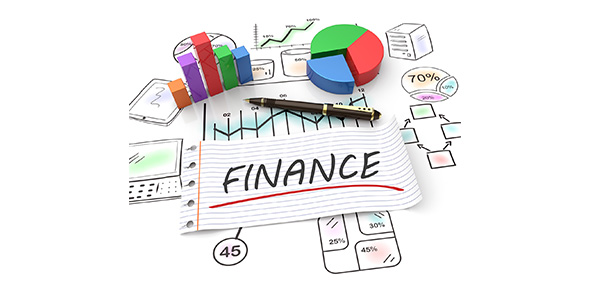# Physical And Chemical Properties And Changes-test A

35 Questions | Total Attempts: 1365SettingsCreate your own QuizIn this scientific quiz, we’ll be looking at a number of physical and chemical properties in objects and how they change, with reference to such topics as volume, matter, litmus paper, density and more. What can you tell us?

• 1.
The amount of matter something has.
• A.

Acid

• B.

Base

• C.

Chemical property

• D.

Mass

• 2.
The amount of space something takes up.
• A.

Volume

• B.

Base

• C.

Chemical property

• D.

Mass

• 3.
A substance that turns blue litmus paper red.
• A.

Acid

• B.

Base

• C.

Chemical property

• D.

Mass

• 4.
A substance that turns red litmus paper blue.
• A.

Acid

• B.

Base

• C.

Chemical property

• D.

Mass

• 5.
Something that describes a substance by itself.
• A.

Physical property

• B.

Base

• C.

Chemical property

• D.

Mass

• 6.
Something that describes the ability of a substance to react with other substances.
• A.

Physical property

• B.

Base

• C.

Chemical property

• D.

Mass

• 7.
A student can't remember how to find the density of an object.  What would you tell him to do to calculate density?
• A.

Find the object's mass and add its volume

• B.

Divide the object's mass by its volume

• C.

Subtract the object's mass from its volume

• D.

Multiply the object's mass by its volume

• 8.
A student is reading the warning on a drain-cleaning product.  It says that the product is a strong base.  What is likely to be the pH of the drain cleaner?
• A.

2

• B.

6

• C.

8

• D.

12

• 9.
A student has a beaker containing a clear liquid.  She puts a drop of this liquid on a piece of blue litmus paper, and the paper turns red. Then she adds 10 mg of baking soda to the beaker and stirs.  Now drops of the liquid do not affect the color of red nor blue litmus paper. What kind of liquid is in the student's beaker now?
• A.

An acid

• B.

A base

• C.

A liquid that is neutral

• D.

Cannot tell

• 10.
Which of the following is not a physical property?
• A.

The ability of a substance to dissolve in water

• B.

The ability of a substance to change directly into gas

• C.

The ability of a substance to react with oxygen

• D.

The ability of a substance to melt at a specific temperature

• 11.
Which of the following is a chemical property?
• A.

Length of a matchstick

• B.

Color of a matchstick

• C.

Ability of a matchstick to float on water

• D.

Ability of a matchstick to burn when struck

• 12.
The element helium (He) will not react chemically with other elements. Which word describes this property of helium?
• A.

Acidic

• B.

Reactive

• C.

Basic

• D.

Stable

• 13.
A substance that turns red litmus paper blue is called a
• A.

Base

• B.

Volume

• C.

Stability

• D.

Indicators

• 14.
The amount of space an object takes up is referred to as its
• A.

Volume

• B.

Stability

• C.

Indicators

• D.

Mass

• 15.
One or more new substances are formed during a
• A.

Chemical change

• B.

Base

• C.

Volume

• D.

Stability

• 16.
The amount of matter an object contains is referred to as its
• A.

Mass

• B.

Chemical change

• C.

Base

• D.

Volume

• 17.
Litmus paper and cabbage juice are
• A.

Indicators

• B.

Mass

• C.

Chemical change

• D.

Base

• 18.
Which of the following is a chemical property?
• A.

Is attracted to magnets

• B.

Burns easily

• C.

Has a volume of 24 L

• D.

Has a smooth surface

• 19.
A board has a mass of 750 g.  If you ground the whole board down to sawdust, what would be the mass of the pile of sawdust?
• A.

Much less than 750 g

• B.

A little less than 750 g

• C.

Exactly 750 g

• D.

More than 750 g

• 20.
Which of the following is a physical property?
• A.

Burns easily

• B.

Does not react with other substances

• C.

Mixes with water

• D.

Reacts with chlorine to form salt

• 21.
Which would be the pH value of a strong base?
• A.

13

• B.

7

• C.

5

• D.

0

• 22.
Which common household item below is the strongest acid?
• A.

Apples-pH of 3

• B.

Milk-pH of 6

• C.

Lemon juice-pH of 2

• D.

Drain cleaner-pH of 12

• 23.
What are the three main states of matter?
• A.

Solid, liquid, and gas

• B.

Ice, water, and steam

• C.

Temperature, pressure, and energy

• D.

Hot, cold, and warm

• 24.
What might happen if you mixed a base with an equal amount of acid?
• A.

You would see an explosive chemical reaction

• B.

The acid would destroy the base

• C.

The base would destroy the acid

• D.

They would balance out and you would have a neutral substance

• 25.
Pure water (H2O) has a pH level of
• A.

3

• B.

7 (neutral)

• C.

9

• D.

11

Related TopicsBack to top
×

Wait!
Here's an interesting quiz for you.